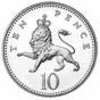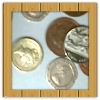#### You may also like### Coin Tossing Games

You and I play a game involving successive throws of a fair coin. Suppose I pick HH and you pick TH. The coin is thrown repeatedly until we see either two heads in a row (I win) or a tail followed by a head (you win). What is the probability that you win?### Win or Lose?

A gambler bets half the money in his pocket on the toss of a coin, winning an equal amount for a head and losing his money if the result is a tail. After 2n plays he has won exactly n times. Has he more money than he started with?A counter is placed in the bottom right hand corner of a grid. You toss a coin and move the star according to the following rules: ... What is the probability that you end up in the top left-hand corner of the grid?

# Same Number!

##### Age 14 to 16Challenge Level

Same Number! poses some really challenging problems and we hope that you enjoyed exploring them.

Here are some of the solutions which have been previously submitted to the team:

Joseph from Wilson's School shared his thoughts about the problem:

I think it is likely that everyone's number will be different, as there are 225 numbers and only 30 people. I expect there will only be a few times when everyone's numbers are different.
Yes, I am surprised by the fact that everyone's number were different only a few times.

In a class of 30, if a teacher asks the class to write down a number from 1 to 225, then the probabilitity of the same number being read out is as follows:

The probability that the $2^{nd}$ student reads out a number that is different to the one read out by the $1^{st}$ student is $\frac{224}{225}$

The chance of a $3{rd}$ student reading out a different number is $\frac{223}{225}$
The probability that the first 3 students will all read out different numbers is $\frac{224}{225}\times\frac{223}{225}$

The chance of a $4^{th}$ student reading out a different number is $\frac{222}{225}$
The probability that the first 4 students will all read out different numbers is $\frac{224}{225}\times\frac{223}{225}\times\frac{222}{225}$

The probability that the whole class will all read out different numbers is $\frac{224}{225}\times\frac{223}{225}\times\frac{222}{225}.......\times\frac{196}{225}$ = 0.13 (to 2 dec. places)

This implies the probability that at least two students have written the same number is
1 - 13% = 87%

-------

In the Birthday Problem we were asked:

How many people do you need in a room so that the chance that there
will be at least two people with the same birthday is greater than 50%?

Imagine the people enter into the room one by one...

The probability that the $2^{nd}$ person to enter does not share their birthday with the $1^{st}$ person is $\frac{364}{365}$= 0.997

The probability that the first three people to enter do not share the same birthday is $\frac{364}{365}\times\frac{363}{365}$= 0.992

If you keep multiplying like this you will eventually find an answer which is just less than 0.50 which happens once 23 people have entered the room:

The probability that the first 23 people to enter do not share the same birthday is
$\frac{364}{365}\times\frac{363}{365}.....\times\frac{343}{365}$ = 0.49

So when there are 23 people in the room, the probability that at least two people share the same birthday is $1- 0.49 = 0.51$

-------

A student presented a very clear explanation of the general calculation process for these types of problem:

The probability P that nobody shares a number/birthday in a given population of x, where n is the maximum number, is:
$$\frac{n(n-1)(n-2)....(n-(x-1))}{n^x}$$
Knowing two of n, x, or P means we can feed in the numbers to find the last variable.

Therefore the probability of two or more people sharing numbers/birthday in a given population of x, where n is the maximum number, is:
$$1-\frac{n(n-1)(n-2)....(n-(x-1))}{n^x}$$

Well done to everyone!